(2 Kb)
Updated: 04-26-11 05:38 AM
Pictures
File Info
 Updated: 04-26-11 05:38 AM Created: 02-13-11 02:19 AM Downloads: 3,880 Favorites: 56 MD5:

# Homing Digeon

Version: 1.3
by: Wobin [More]

I got really tired of having to flip back and forth between the World Map and the Flight Map to find the closest flightpoint for all the archaeology digs, so this addon merely overlays the digs onto the flight map directly, so it's easier to pick destinations to idlefly to.

Thanks to karl_w_w on #wowuidev IRC for the name

1.3 - Improved accuracy between flightmap and world map on each continent
1.2 - Removed shovel enlargement. Added in 'closest flightpoint' indicator
1.1 - Made the shovels larger, cleaned up the code to hide all shovels between continents

1.0.1 - No code changes, just folder names to be appropriate for the addon name, thanks Sniksder for pointing that out
Optional Files (0)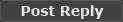Comment Options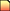12-08-14, 10:52 PM menace97 Premium Member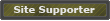Forum posts: 4 File comments: 118 Uploads: 0 thank you very much Gello! this is such a handy addon. i really appreciate your help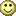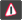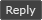12-08-14, 07:27 PM
Gello
 Originally Posted by menace97 just out of curiosity Gello, is that comma after "Kalimdor" intentional? it just looked odd being the only one with a comma after it. thanks so much for keeping this alive Gello *bows*
Oops nah it's not intentional. I started to rewrite the comment as
 --  - Kalimdor,  - Eastern Kingdoms,  - etc
But decided afterwards to keep the same formatting as the original. I just forgot to remove a ,.

Incidentally, those new magic numbers aren't perfect. I don't have any method of determining them other than trial and error. If anyone comes up with better numbers by all means feel free to post them.

The taxi map is (generally) only a portion of the continent's map. So the x/y scale is the ratio of the displayed taxi continent vs the displayed world map continent. And the x/y offset is how far to nudge after scaling.12-07-14, 05:13 AM menace97 Premium MemberForum posts: 4 File comments: 118 Uploads: 0 just out of curiosity Gello, is that comma after "Kalimdor" intentional? it just looked odd being the only one with a comma after it. thanks so much for keeping this alive Gello *bows* Last edited by menace97 : 12-07-14 at 05:24 AM.11-26-14, 05:53 AM
tednik
 Originally Posted by Gello For WoD, change this: Code: ```local x1, x2, y1, y2 = WorldMap_GetPOITextureCoords(177) local digSites = {} -- Turns out every flightmap and atlas map for each continent have differing -- ratios between them. So magic numbers ahoy --  - Kalimdor --  - Eastern Kingdoms --  - Outlands --  - Northrend local MagicXRatio = {  = 1.2,  = 1.3,  = 1.3,  = 1.5 } local MagicYRatio = {  = 0.8,  = 1,  = 1,  = 1} local MagicYOffset = {  = -30,  = 5,  = -5,  = -5} local MagicXOffset = {  = 15,  = 0,  = 0,  = 0 }``` to this: Code: ```local x1, x2, y1, y2 = GetPOITextureCoords(177) local digSites = {} -- Turns out every flightmap and atlas map for each continent have differing -- ratios between them. So magic numbers ahoy --  - Kalimdor, --  - Eastern Kingdoms --  - Outlands --  - Northrend --  - Maelstrom --  - Pandaria --  - Draenor local MagicXRatio = {  = 1.2,  = 1.3,  = 1.3,  = 1.5, =1, =1.3, =1.35 } local MagicYRatio = {  = 0.8,  = 1,  = 1,  = 1, =1, =.875, =0.9 } local MagicYOffset = {  = -30,  = 5,  = -5,  = -5, =0, =0, =0 } local MagicXOffset = {  = 15,  = 0,  = 0,  = 0, =0, =-10, =36 }```
tyvm for this11-23-14, 03:46 AM Gello A Firelord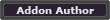Forum posts: 492 File comments: 543 Uploads: 64 For WoD, change this: Code: ```local x1, x2, y1, y2 = WorldMap_GetPOITextureCoords(177) local digSites = {} -- Turns out every flightmap and atlas map for each continent have differing -- ratios between them. So magic numbers ahoy --  - Kalimdor --  - Eastern Kingdoms --  - Outlands --  - Northrend local MagicXRatio = {  = 1.2,  = 1.3,  = 1.3,  = 1.5 } local MagicYRatio = {  = 0.8,  = 1,  = 1,  = 1} local MagicYOffset = {  = -30,  = 5,  = -5,  = -5} local MagicXOffset = {  = 15,  = 0,  = 0,  = 0 }``` to this: Code: ```local x1, x2, y1, y2 = GetPOITextureCoords(177) local digSites = {} -- Turns out every flightmap and atlas map for each continent have differing -- ratios between them. So magic numbers ahoy --  - Kalimdor, --  - Eastern Kingdoms --  - Outlands --  - Northrend --  - Maelstrom --  - Pandaria --  - Draenor local MagicXRatio = {  = 1.2,  = 1.3,  = 1.3,  = 1.5, =1, =1.3, =1.35 } local MagicYRatio = {  = 0.8,  = 1,  = 1,  = 1, =1, =.875, =0.9 } local MagicYOffset = {  = -30,  = 5,  = -5,  = -5, =0, =0, =0 } local MagicXOffset = {  = 15,  = 0,  = 0,  = 0, =0, =-10, =36 }```10-20-14, 09:08 AM Saintgabrial A Kobold Labourer Forum posts: 0 File comments: 35 Uploads: 0 Needs a MoP and WoD Update I realy miss this addon. I wish we could get an update. Fan or otherwise!10-19-14, 10:07 AM
Draven006
A Fallenroot Satyr

Forum posts: 23

 Originally Posted by Gello In addition to the change in line 3 mentioned earlier (change WorldMap_GetPOITextureCoords to GetPOITextureCoords), replace these lines: Code: ```local MagicXRatio = {  = 1.2,  = 1.3,  = 1.3,  = 1.5 } local MagicYRatio = {  = 0.8,  = 1,  = 1,  = 1} local MagicYOffset = {  = -30,  = 5,  = -5,  = -5} local MagicXOffset = {  = 15,  = 0,  = 0,  = 0 }``` with these lines: Code: ```local MagicXRatio = {  = 1.2,  = 1.3,  = 1.3,  = 1.5,  = 1.3,  = 1.0 } local MagicYRatio = {  = 0.8,  = 1,  = 1,  = 1,  = 0.875,  = 1.0 } local MagicXOffset = {  = 15,  = 0,  = 0,  = 0,  = 30,  = 0 } local MagicYOffset = {  = -30,  = 5,  = -5,  = -5,  = 0,  = 0 }``` and the addon should be current for MoP.
This worked to a point, so that was good.
How did you work out what the Ratio and offsets were? Might be nice to help out for WoD :-)07-15-13, 08:50 PM Gello A FirelordForum posts: 492 File comments: 543 Uploads: 64 Re: MOP Update! please In addition to the change in line 3 mentioned earlier (change WorldMap_GetPOITextureCoords to GetPOITextureCoords), replace these lines: Code: ```local MagicXRatio = {  = 1.2,  = 1.3,  = 1.3,  = 1.5 } local MagicYRatio = {  = 0.8,  = 1,  = 1,  = 1} local MagicYOffset = {  = -30,  = 5,  = -5,  = -5} local MagicXOffset = {  = 15,  = 0,  = 0,  = 0 }``` with these lines: Code: ```local MagicXRatio = {  = 1.2,  = 1.3,  = 1.3,  = 1.5,  = 1.3,  = 1.0 } local MagicYRatio = {  = 0.8,  = 1,  = 1,  = 1,  = 0.875,  = 1.0 } local MagicXOffset = {  = 15,  = 0,  = 0,  = 0,  = 30,  = 0 } local MagicYOffset = {  = -30,  = 5,  = -5,  = -5,  = 0,  = 0 }``` and the addon should be current for MoP.10-12-12, 09:19 PM Saintgabrial A Kobold Labourer Forum posts: 0 File comments: 35 Uploads: 0 MOP Update! please I love this add-on and no other does what it does. I sure hope someone would update this one. Either a fan update if aloud or something. Please.10-11-12, 06:24 PM kittykatmax A Fallenroot Satyr Forum posts: 21 File comments: 229 Uploads: 0 Any chance for an official update? /begs09-09-12, 06:19 PM
Gello
Re: Reciving Error with 5.0.4

Change line 3 from:
Code:
`local x1, x2, y1, y2 = WorldMap_GetPOITextureCoords(177)`
to this:
Code:
`local x1, x2, y1, y2 = GetPOITextureCoords(177)`
Seems to be only change needed until Pandaria, where new coefficients will be needed.

 Originally Posted by nickyjean Interface\AddOns\HomingDigeon\HomingDigeon.lua:3: attempt to call global 'WorldMap_GetPOITextureCoords' (a nil value) Count: 1 Call Stack: [C]: in function `WorldMap_GetPOITextureCoords' Interface\AddOns\HomingDigeon\HomingDigeon.lua:3: in main chunk08-29-12, 06:53 PM nickyjean Premium MemberForum posts: 283 File comments: 118 Uploads: 0 Reciving Error with 5.0.4 Interface\AddOns\HomingDigeon\HomingDigeon.lua:3: attempt to call global 'WorldMap_GetPOITextureCoords' (a nil value) Count: 1 Call Stack: [C]: in function `WorldMap_GetPOITextureCoords' Interface\AddOns\HomingDigeon\HomingDigeon.lua:3: in main chunk02-13-11, 10:25 AM
Wobin
 Originally posted by sniksder You have to remove the hypen between homing and digeon before the game will let this work.
Sorry about that, fixed the package
__________________
Wob's Portal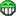02-13-11, 10:00 AM
sniksder
A Kobold Labourer

Forum posts: 1
 Originally posted by nightcracker This is a great idea!
You have to remove the hypen between homing and digeon before the game will let this work.
Last edited by sniksder : 02-13-11 at 10:17 AM.02-13-11, 04:27 AM nightcracker A Molten GiantForum posts: 716 File comments: 428 Uploads: 22 This is a great idea! __________________ Three things are certain, Death, taxes and site not found, You, victim of one.Report this File

Category Jump: